Next: The Staggered Leapfrog Method Up: Initial Conditions on the Previous: The Initial value of

We noted in section 6.3.3 that the time dependence of the modes comes from their explicit time dependence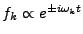, from factors of the scale factor, and from the time dependence of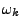itself. Using program variables for the fields the time dependence of the modes is given by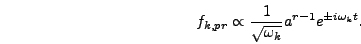(6.92)

Thus the derivative is given by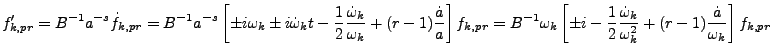(6.93)

where the last step uses the fact that initially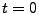and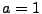. Neglecting the time dependence ofas we did earlier amounts to making the approximation(6.94)

which is precisely the condition thatis changing adiabatically. If this condition is not satisfied in the late stages of inflation then gravitational particle production will occur and it will no longer make sense to take the vacuum fluctuations of equation (6.51) as initial conditions.

There's another way to view this condition. Gravitational particle production will occur unless. Since this condition is automatically satisfied forconsider the opposite case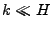, for which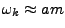. Then neglecting the time dependence of,when, so the conditionis equivalent to the condition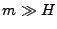. In factis the stronger (and more accurate) condition because it also specifies thatshouldn't be changing rapidly, which would lead to particle production irrespective of the value of. However, all particle masses should vary slowly during inflation because they should only depend on constants and on the value of the inflaton, which must be changing slowly.

In the case of a field with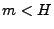during inflation the approximation that the field ends inflation in its ground state is no longer valid. In the limitthe fluctuations of the field produced during inflation can be accurately described by Hankel functions . However in this case the fields will be copiously produced during inflation, leading to severe cosmological problems . For this reason we do not implement these Hankel function solutions in the lattice program. In order to avoid the moduli problem associated with light fields it's best to assume that some mechanism must have given all scalar fields large masses during inflation, in which case equation (6.51) is an accurate expression for the modes at the end of inflation.Next: The Staggered Leapfrog Method Up: Initial Conditions on the Previous: The Initial value of